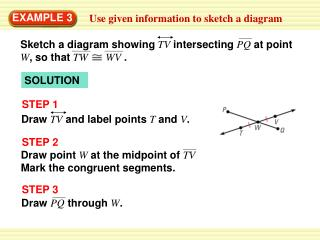DownloadDownload PresentationEXAMPLE 3

# EXAMPLE 3

Download Presentation## EXAMPLE 3

- - - - - - - - - - - - - - - - - - - - - - - - - - - E N D - - - - - - - - - - - - - - - - - - - - - - - - - - -
##### Presentation Transcript

1. Sketch a diagram showing TVintersecting PQat point W, so that TWWV. Draw TVand label points Tand V. Draw point Wat the midpoint of TVMark the congruent segments. Draw PQthrough W. EXAMPLE 3 Use given information to sketch a diagram SOLUTION STEP 1 STEP 2 STEP 3

2. Which of the following statements cannot be assumed from the diagram? ABplane S CDplane T AFintersects BCat point B. No drawn line connects E, B, and D, so you cannot assume they are collinear. With no right angle marked, you cannot assume CDplane T. EXAMPLE 4 Interpret a diagram in three dimensions A, B, and Fare collinear. E, B, and Dare collinear. SOLUTION

3. 3. If the given information stated PWand QWare congruent, how would you indicate that in the diagram? ANSWER for Examples 3 and 4 GUIDED PRACTICE In Exercises 3 and 4, refer back to Example 3.

4. 4. Name a pair of supplementary angles in the diagram. Explain. ANSWER In the diagram the angle TWP and VWP form a linear pair So, angle TWP, VWP are a pair of supplementary angles. for Examples 3 and 4 GUIDED PRACTICE

5. 5. In the diagram for Example 4, can you assume plane Sintersects plane Tat BC? ANSWER Yes for Examples 3 and 4 GUIDED PRACTICE

6. 6. Explain how you know that ABBCin Example 4. ANSWER It is given that line AB is perpendicular to plane S, therefore line AB is perpendicular to every line in the plane that intersect it at point B. So, AB BC for Examples 3 and 4 GUIDED PRACTICE